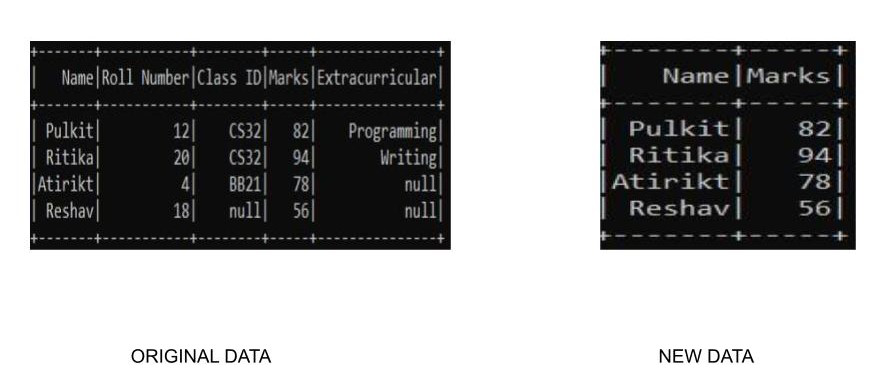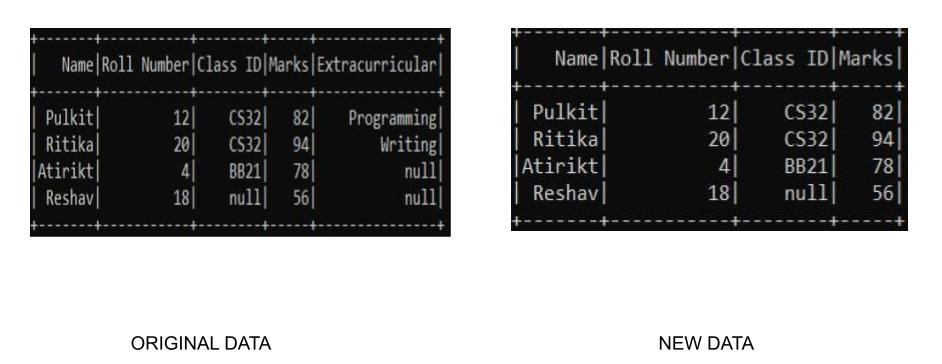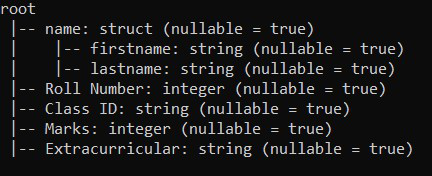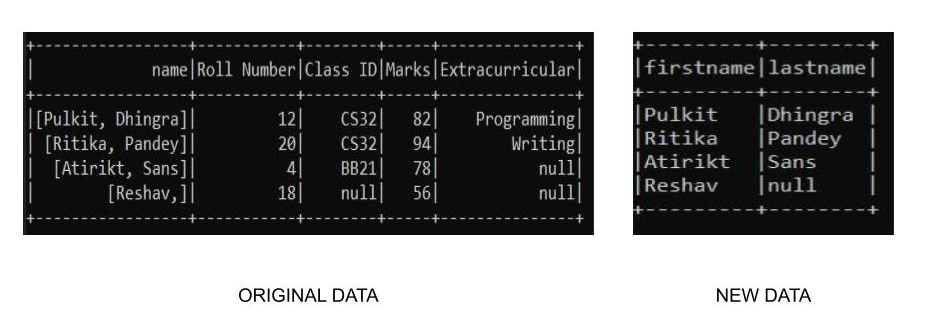# Select columns in PySpark dataframe

• Last Updated : 08 May, 2021

In this article, we will learn how to select columns in PySpark dataframe.

### Function used:

In PySpark we can select columns using the select() function. The select() function allows us to select single or multiple columns in different formats.

Syntax: dataframe_name.select( columns_names )

Note: We are specifying our path to spark directory using the findspark.init() function in order to enable our program to find the location of apache spark in our local machine. Ignore this line if you are running the program on cloud. Suppose we have our spark folder in c drive by name of spark so the function would look something like: findspark.init(‘c:/spark’). Not specifying the path sometimes may lead to py4j.protocol.Py4JError error when running the program locally.

Example 1: Select single or multiple columns

We can select single or multiple columns using the select() function by specifying the particular column name. Here we are using our custom dataset thus we need to specify our schema along with it in order to create the dataset.

## Python3

 `# select single and multiple columns``from` `pyspark.sql.types ``import` `StructType, StructField, StringType, IntegerType, FloatType``from` `pyspark.sql ``import` `SparkSession``import` `findspark`` ` ` ` `findspark.init(``'c:/spark'``)`` ` `# Initialize our data``data2 ``=` `[(``"Pulkit"``, ``12``, ``"CS32"``, ``82``, ``"Programming"``),``         ``(``"Ritika"``, ``20``, ``"CS32"``, ``94``, ``"Writing"``),``         ``(``"Atirikt"``, ``4``, ``"BB21"``, ``78``, ``None``),``         ``(``"Reshav"``, ``18``, ``None``, ``56``, ``None``)``         ``]`` ` `# Start spark session``spark ``=` `SparkSession.builder.appName(``"Student_Info"``).getOrCreate()`` ` `# Define schema``schema ``=` `StructType([``    ``StructField(``"Name"``, StringType(), ``True``),``    ``StructField(``"Roll Number"``, IntegerType(), ``True``),``    ``StructField(``"Class ID"``, StringType(), ``True``),``    ``StructField(``"Marks"``, IntegerType(), ``True``),``    ``StructField(``"Extracurricular"``, StringType(), ``True``)``])`` ` `# read the dataframe``df ``=` `spark.createDataFrame(data``=``data2, schema``=``schema)`` ` `# slelct columns``df.select(``"Name"``, ``"Marks"``).show()`` ` `# stop the session``spark.stop()`

Output:Note: There are a lot of ways to specify the column names to the select() function. Here we used “column_name” to specify the column. Other ways include (All the examples as shown with reference to the above code):

• df.select(df.Name,df.Marks)
• df.select(df[“Name”],df[“Marks”])
• We can use col() function from pyspark.sql.functions module to specify the particular columns

## Python3

 `from` `pyspark.sql.functions ``import` `col`` ` `df.select(col(``"Name"``),col(``"Marks"``)).show()`

Note: All the above methods will yield the same output as above

Example 2: Select columns using indexing

Indexing provides an easy way of accessing columns inside a dataframe. Indexing starts from 0 and has total n-1 numbers representing each column with 0 as first and n-1 as last nth column. We can use df.columns to access all the columns and use indexing to pass in the required columns inside a select function. Here is how the code will look like. We are using our custom dataset thus we need to specify our schema along with it in order to create the dataset.

## Python3

 `# select spark``from` `pyspark.sql.types ``import` `StructType, StructField, StringType, IntegerType, FloatType``from` `pyspark.sql ``import` `SparkSession``import` `findspark`` ` ` ` `findspark.init(``'c:/spark'``)`` ` `# Initialize our data``data2 ``=` `[(``"Pulkit"``, ``12``, ``"CS32"``, ``82``, ``"Programming"``),``         ``(``"Ritika"``, ``20``, ``"CS32"``, ``94``, ``"Writing"``),``         ``(``"Atirikt"``, ``4``, ``"BB21"``, ``78``, ``None``),``         ``(``"Reshav"``, ``18``, ``None``, ``56``, ``None``)``         ``]`` ` `# Start spark session``spark ``=` `SparkSession.builder.appName(``"Student_Info"``).getOrCreate()`` ` `# Define schema``schema ``=` `StructType([``    ``StructField(``"Name"``, StringType(), ``True``),``    ``StructField(``"Roll Number"``, IntegerType(), ``True``),``    ``StructField(``"Class ID"``, StringType(), ``True``),``    ``StructField(``"Marks"``, IntegerType(), ``True``),``    ``StructField(``"Extracurricular"``, StringType(), ``True``)``])`` ` `# read the dataframe``df ``=` `spark.createDataFrame(data``=``data2, schema``=``schema)`` ` `# select the columns``df.select(df.columns[:``4``]).show()`` ` `# stop session``spark.stop()`

Output:Example 3: Access nested columns of a dataframe

While creating a dataframe there might be a table where we have nested columns like, in a column name “Marks” we may have sub-columns of Internal or external marks, or we may have separate columns for the first middle, and last names in a column under the name. In order to access the nested columns inside a dataframe using the select() function, we can specify the sub-column with the associated column. Here we are using our custom dataset thus we need to specify our schema along with it in order to create the dataset.

## Python3

 `# findspark``from` `pyspark.sql.types ``import` `StructType, StructField, StringType, IntegerType, FloatType``from` `pyspark.sql ``import` `SparkSession``import` `findspark`` ` ` ` `findspark.init(``'c:/spark'``)`` ` `# initialize the data``data ``=` `[``    ``((``"Pulkit"``, ``"Dhingra"``), ``12``, ``"CS32"``, ``82``, ``"Programming"``),``    ``((``"Ritika"``, ``"Pandey"``), ``20``, ``"CS32"``, ``94``, ``"Writing"``),``    ``((``"Atirikt"``, ``"Sans"``), ``4``, ``"BB21"``, ``78``, ``None``),``    ``((``"Reshav"``, ``None``), ``18``, ``None``, ``56``, ``None``)``]`` ` `# start spark session``spark ``=` `SparkSession.builder.appName(``"Student_Info"``).getOrCreate()`` ` `# initialize the schema of the data``schema ``=` `StructType([``    ``StructField(``'name'``, StructType([``        ``StructField(``'firstname'``, StringType(), ``True``),``        ``StructField(``'lastname'``, StringType(), ``True``)``    ``])),``    ``StructField(``"Roll Number"``, IntegerType(), ``True``),``    ``StructField(``"Class ID"``, StringType(), ``True``),``    ``StructField(``"Marks"``, IntegerType(), ``True``),``    ``StructField(``"Extracurricular"``, StringType(), ``True``)``])``# create a dataframe``df2 ``=` `spark.createDataFrame(data``=``data, schema``=``schema)`` ` `# display the schema``df2.printSchema()`` ` `# select operation``df2.select(``"name.firstname"``, ``"name.lastname"``).show(truncate``=``False``)`` ` `# stop session``spark.stop()`

Output:

Here we can se we have a dataset of following schemaWe have a column name with sub columns as firstname and lastname. Now as we performed the select operation we have an output likeMy Personal Notes arrow_drop_up